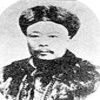#### You may also like### Very Old Man

Is the age of this very old man statistically believable?### bioNRICH

bioNRICH is the area of the stemNRICH site devoted to the mathematics underlying the study of the biological sciences, designed to help develop the mathematics required to get the most from your study of biology at A-level and university.### Catalyse That!

Can you work out how to produce the right amount of chemical in a temperature-dependent reaction?

# Predator - Prey Systems

##### Age 16 to 18Challenge Level

In many eco-systems there exists a main carnivorous predator which has a single type of herbivorous prey as its food source. Clearly, the main risk to the predators' expansion is a reduction in the supply of its food source, whereas in a fertile environment the main risk to the herbivore is a rise in the number of carnivores.

We can model such sytems rather effectively using differential equations.

Let us suppose that the population of carnivores at a time $t$ is $C(t)$ and the population of herbivores is $H(t)$. Clearly these variable change over time and are interdependent. For example, an increase in the population of carnovores will quickly lead to a decrease in the number of herbivores. If there were no carnivores then the herbivores would rapidly grow in number, whereas if there were no herbivores then the carnivores would soon die out.

It is suggested that, for positive constants $a, b, c, d$ a pair of equations of the following form would provide be a good model
$$\frac{dC}{dt} = \pm a C(t) \pm b C(t)H(t) \quad\quad \frac{dH}{dt} = \pm c H(t) \pm d C(t)H(t)$$
What signs would you need to choose in each case, why might each term be present and what would be the interpretation of each constant?

Mathematically how would we encode either of the species becoming extinct? Is there a possible steady state of numbers?

Make up some numbers which seem sensible. What happens as your model evolves? Is it possible, impossible or certain that either species becomes extinct?

How might you modify your equations for a system consisting of two different types of herbivore competing for the same, limited food supply (such as on a small island)?